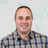Foundation

## Foundation Forum

##### Error in function calc-em
• 2
Replies

Hi,

the calc-em function will be used for calculating the values for the breakpoints. But it seems there is an error.

```@function em-calc(\$values, \$base-value: \$rem-base) {
// is this line correct?
//\$remValues: rem-calc(\$values, \$base-value: \$rem-base);
// changed to this:
\$remValues: rem-calc(\$values, \$base-value);
\$max: length(\$remValues);

@if \$max == 1 { @return strip-unit(nth(\$remValues, 1)) * 1em; }

\$emValues: ();
@for \$i from 1 through \$max {
\$emValues: append(\$emValues, strip-unit(nth(\$remValues, \$i)) * 1em);
}
@return \$emValues;
}

```

is this line correct? \$remValues: rem-calc(\$values, \$base-value: \$rem-base);

if a call this: em-calc(320,16) then in the function the rem-calc function calculates with 14 and not with 16. It should calculate with 16. Thats why i changed this line. Is this an error or why uses the rem-calc function not the correct value?

• 2
Replies

Hi,

the calc-em function will be used for calculating the values for the breakpoints. But it seems there is an error.

```@function em-calc(\$values, \$base-value: \$rem-base) {
// is this line correct?
//\$remValues: rem-calc(\$values, \$base-value: \$rem-base);
// changed to this:
\$remValues: rem-calc(\$values, \$base-value);
\$max: length(\$remValues);

@if \$max == 1 { @return strip-unit(nth(\$remValues, 1)) * 1em; }

\$emValues: ();
@for \$i from 1 through \$max {
\$emValues: append(\$emValues, strip-unit(nth(\$remValues, \$i)) * 1em);
}
@return \$emValues;
}

```

is this line correct? \$remValues: rem-calc(\$values, \$base-value: \$rem-base);

if a call this: em-calc(320,16) then in the function the rem-calc function calculates with 14 and not with 16. It should calculate with 16. Thats why i changed this line. Is this an error or why uses the rem-calc function not the correct value?

#####Rafi Benkualabout 4 years ago

What's your base font size? The default is 16 but if you changed it that would affect the output of this function.

#####Alexabout 4 years ago

Hi,

my base font size is 14px, but for the breakpoints i have to use 16px as the base, because breakpoints in em are based on the browser standard of 16px, no matter what the base font size is.

what i don't inderstand is this line
\$remValues: rem-calc(\$values, \$base-value: \$rem-base);

In the function em-calc i can use a param for the base-value and why is this value not used by the rem-calc function? What is the point of the variable \$base-value? This variable will never be used in this function. Breakpoints are not calculated correct if the standard of 16px will be changed.